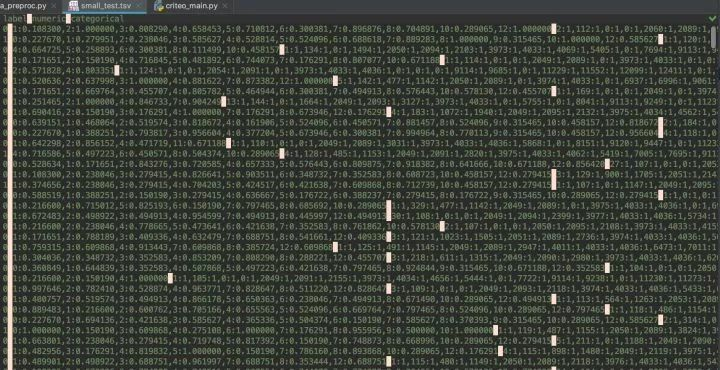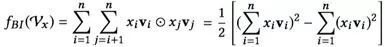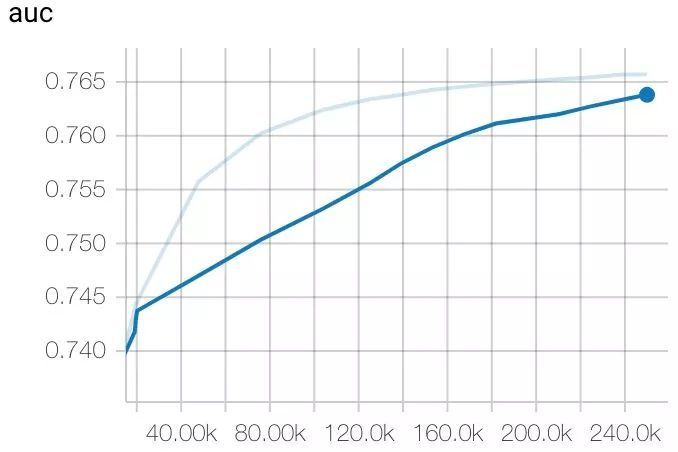# 如何实现支持多值、稀疏、共享权重的 DeepFM## 缘起

DeepFM 不算什么新技术了，用 TensorFlow 实现 DeepFM 也有开源实现，那我为什么要炒这个冷饭，重复造轮子？

• 每个 field 都使用独立的 embedding 矩阵来映射 app 向量，整个模型需要优化的变量是共享权重模型的 3 倍，既耗费了更多的计算资源，也容易导致过拟合。
• 每个 field 的稀疏程度是不一样的，同一个 app，在“活跃列表”中出现得更频繁，其 embedding 向量就有更多的训练机会，而在“卸载列表”中较少出现，其 embedding 向量得不到足够训练，恐怕最后与随机初始化无异。

• 减小优化变量的数目，既节省计算资源，又减轻“过拟合”风险
• 同一个 embedding 矩阵，为多个 field 提供映射向量，类似于“多任务学习”，使每个 embedding 向量得到更多的训练机会，同时也要满足多个 field 的需求（比如同一个 app 的向量，既要体现‘经常使用它’对 y 的影响，也要体现‘卸载它’对 y 值的影响），也降低了“过拟合”的风险。

## 数据预处理

• 这种处理方法，不是为了提高 criteo 数据集上的模型性能，只是为了模拟实际系统中将会遇到的“一列多值”和“稀疏”数据集。接下来会看到，DeepFM 中，FM 中的二阶交叉，不会受拆分成两个 field 的影响。受影响的主要是 Deep 侧的输入层，详情见”DNN 预测部分”一节 。
• 另外，criteo 数据集无法演示“权重共享”的功能。

• 数值特征，因为多数表示 " 次数 "，因此先做了一个 log 变化，减弱长尾数据的影响，再做了一个 min/max scaling，毕竟底层还是线性算法，要排除特征间不同 scale 的影响。注意，千万不能做“zero mean, unit variance”的 standardize，因为那样会破坏数据的稀疏性。
• 类别特征，剔除了一些生僻的 tag，建立字典，将原始数据中的字符串 tag 转化为整数的 index## input_fn

• 网上能够搜到的 TensorFlow 读文本文件的代码，都是读“每列只有一个值的 csv”这样规则的数据格式。但是，上图所示的数据，却非常不规则，每行先是由“\t”分隔，第列中再由“,”分隔成数目不同的“键值对”，每个‘键值对’再由“:”分隔。
• 我希望提供给 model 稀疏矩阵，方便 model 中排零计算，提升效率。

def _decode_tsv(line):    columns = tf.decode_csv(line, record_defaults=DEFAULT_VALUES, field_delim='\t')    y = columns     feat_columns = dict(zip((t for t in COLUMNS_MAX_TOKENS), columns[1:]))    X = {}    for colname, max_tokens in COLUMNS_MAX_TOKENS:        # 调用 string_split 时，第一个参数必须是一个 list，所以要把 columns[colname] 放在 [] 中        # 这时每个 kv 还是'k:v'这样的字符串        kvpairs = tf.string_split([feat_columns[colname]], ',').values[:max_tokens]         # k,v 已经拆开, kvpairs 是一个 SparseTensor，因为每个 kvpair 格式相同，都是 "k:v"        # 既不会出现 "k"，也不会出现 "k:v1:v2:v3:..."        # 所以，这时的 kvpairs 实际上是一个满阵        kvpairs = tf.string_split(kvpairs, ':')         # kvpairs 是一个 [n_valid_pairs,2] 矩阵        kvpairs = tf.reshape(kvpairs.values, kvpairs.dense_shape)         feat_ids, feat_vals = tf.split(kvpairs, num_or_size_splits=2, axis=1)        feat_ids = tf.string_to_number(feat_ids, out_type=tf.int32)        feat_vals = tf.string_to_number(feat_vals, out_type=tf.float32)         # 不能调用 squeeze, squeeze 的限制太多, 当原始矩阵有 1 行或 0 行时，squeeze 都会报错        X[colname + "_ids"] = tf.reshape(feat_ids, shape=[-1])        X[colname + "_values"] = tf.reshape(feat_vals, shape=[-1])     return X, y

 def input_fn(data_file, n_repeat, batch_size, batches_per_shuffle):    # ----------- prepare padding    pad_shapes = {}    pad_values = {}    for c, max_tokens in COLUMNS_MAX_TOKENS:        pad_shapes[c + "_ids"] = tf.TensorShape([max_tokens])        pad_shapes[c + "_values"] = tf.TensorShape([max_tokens])         pad_values[c + "_ids"] = -1  # 0 is still valid token-id, -1 for padding        pad_values[c + "_values"] = 0.0     # no need to pad labels    pad_shapes = (pad_shapes, tf.TensorShape([]))    pad_values = (pad_values, 0)     # ----------- define reading ops    dataset = tf.data.TextLineDataset(data_file).skip(1)  # skip the header    dataset = dataset.map(_decode_tsv, num_parallel_calls=4)     if batches_per_shuffle > 0:        dataset = dataset.shuffle(batches_per_shuffle * batch_size)     dataset = dataset.repeat(n_repeat)    dataset = dataset.padded_batch(batch_size=batch_size,                                   padded_shapes=pad_shapes,                                   padding_values=pad_values)     iterator = dataset.make_one_shot_iterator()    dense_Xs, ys = iterator.get_next()     # ----------- convert dense to sparse    sparse_Xs = {}    for c, _ in COLUMNS_MAX_TOKENS:        for suffix in ["ids", "values"]:            k = "{}_{}".format(c, suffix)            sparse_Xs[k] = tf_utils.to_sparse_input_and_drop_ignore_values(dense_Xs[k])     # ----------- return    return sparse_Xs, ys

## 建立共享权重

• field 就是active_pkgs、install_pkgs、uninstall_pkgs这些大类，是 DataFrame 中的每一列
• feature 就是每个 field 下包含的具体内容，一个 field 下允许存在多个 feature，比如前面提到的 qq, weixin, taobao 这样的 app 名称。
• vocabulary 对应例子中的“package 字典”。不同 field 下的 feature 可以来自同一个 vocabulary，即若干 field 共享 vocabulary

• 一个 vocab 对应两个 embedding 矩阵，一个对应 FM 中的线性部分的权重，另一个对应 FM 与 DNN 共享的隐向量（用于二阶与高阶交叉）。
• 所有 embedding 矩阵，以”字典名”存入 dict。不同 field 只要指定相同的“字典名”，就可以共享同一套 embedding 矩阵。

 class EmbeddingTable:    def __init__(self):        self._weights = {}     def add_weights(self, vocab_name, vocab_size, embed_dim):        """        :param vocab_name: 一个 field 拥有两个权重矩阵，一个用于线性连接，另一个用于非线性（二阶或更高阶交叉）连接        :param vocab_size: 字典总长度        :param embed_dim: 二阶权重矩阵 shape=[vocab_size, order2dim]，映射成的 embedding                          既用于接入 DNN 的第一屋，也是用于 FM 二阶交互的隐向量        :return: None        """        linear_weight = tf.get_variable(name='{}_linear_weight'.format(vocab_name),                                        shape=[vocab_size, 1],                                        initializer=tf.glorot_normal_initializer(),                                        dtype=tf.float32)         # 二阶（FM）与高阶（DNN）的特征交互，共享 embedding 矩阵        embed_weight = tf.get_variable(name='{}_embed_weight'.format(vocab_name),                                       shape=[vocab_size, embed_dim],                                       initializer=tf.glorot_normal_initializer(),                                       dtype=tf.float32)         self._weights[vocab_name] = (linear_weight, embed_weight)     def get_linear_weights(self, vocab_name): return self._weights[vocab_name]     def get_embed_weights(self, vocab_name): return self._weights[vocab_name]  def build_embedding_table(params):    embed_dim = params['embed_dim']  # 必须有统一的 embedding 长度     embedding_table = EmbeddingTable()    for vocab_name, vocab_size in params['vocab_sizes'].items():        embedding_table.add_weights(vocab_name=vocab_name, vocab_size=vocab_size, embed_dim=embed_dim)     return embedding_table

## 线性预测部分

 def output_logits_from_linear(features, embedding_table, params):    field2vocab_mapping = params['field_vocab_mapping']    combiner = params.get('multi_embed_combiner', 'sum')     fields_outputs = []    # 当前 field 下有一系列的 <tag:value> 对，每个 tag 对应一个 bias（待优化），    # 将所有 tag 对应的 bias，按照其 value 进行加权平均，得到这个 field 对应的 bias    for fieldname, vocabname in field2vocab_mapping.items():        sp_ids = features[fieldname + "_ids"]        sp_values = features[fieldname + "_values"]         linear_weights = embedding_table.get_linear_weights(vocab_name=vocabname)         # weights: [vocab_size,1]        # sp_ids: [batch_size, max_tags_per_example]        # sp_weights: [batch_size, max_tags_per_example]        # output: [batch_size, 1]        output = embedding_ops.safe_embedding_lookup_sparse(linear_weights, sp_ids, sp_values,                                                            combiner=combiner,                                                            name='{}_linear_output'.format(fieldname))         fields_outputs.append(output)     # 因为不同 field 可以共享同一个 vocab 的 linear weight，所以将各个 field 的 output 相加，会损失大量的信息    # 因此，所有 field 对应的 output 拼接起来，反正每个 field 的 output 都是 [batch_size,1]，拼接起来，并不占多少空间    # whole_linear_output: [batch_size, total_fields]    whole_linear_output = tf.concat(fields_outputs, axis=1)    tf.logging.info("linear output, shape={}".format(whole_linear_output.shape))     # 再映射到 final logits（二分类，也是 [batch_size,1]）    # 这时，就不要用任何 activation 了，特别是 ReLU    return tf.layers.dense(whole_linear_output, units=1, use_bias=True, activation=None)

## 二阶交互预测部分def output_logits_from_bi_interaction(features, embedding_table, params):    field2vocab_mapping = params['field_vocab_mapping']     # 论文上的公式就是要求 sum，而且我也试过 mean 和 sqrtn，都比用 mean 要差上很多    # 但是，这种情况，仅仅是针对 criteo 数据的，还是理论上就必须用 sum，而不能用 mean 和 sqrtn    # 我还不太确定，所以保留一个接口能指定其他 combiner 的方法    combiner = params.get('multi_embed_combiner', 'sum')     # 见《Neural Factorization Machines for Sparse Predictive Analytics》论文的公式 (4)    fields_embeddings = []    fields_squared_embeddings = []     for fieldname, vocabname in field2vocab_mapping.items():        sp_ids = features[fieldname + "_ids"]        sp_values = features[fieldname + "_values"]         # --------- embedding        embed_weights = embedding_table.get_embed_weights(vocabname)        # embedding: [batch_size, embed_dim]        embedding = embedding_ops.safe_embedding_lookup_sparse(embed_weights, sp_ids, sp_values,                                                               combiner=combiner,                                                               name='{}_embedding'.format(fieldname))        fields_embeddings.append(embedding)         # --------- square of embedding        squared_emb_weights = tf.square(embed_weights)         squared_sp_values = tf.SparseTensor(indices=sp_values.indices,                                            values=tf.square(sp_values.values),                                            dense_shape=sp_values.dense_shape)         # squared_embedding: [batch_size, embed_dim]        squared_embedding = embedding_ops.safe_embedding_lookup_sparse(squared_emb_weights, sp_ids, squared_sp_values,                                                                       combiner=combiner,                                                                       name='{}_squared_embedding'.format(fieldname))        fields_squared_embeddings.append(squared_embedding)     # calculate bi-interaction    sum_embedding_then_square = tf.square(tf.add_n(fields_embeddings))  # [batch_size, embed_dim]    square_embedding_then_sum = tf.add_n(fields_squared_embeddings)  # [batch_size, embed_dim]    bi_interaction = 0.5 * (sum_embedding_then_square - square_embedding_then_sum)  # [batch_size, embed_dim]    tf.logging.info("bi-interaction, shape={}".format(bi_interaction.shape))     # calculate logits    logits = tf.layers.dense(bi_interaction, units=1, use_bias=True, activation=None)     # 因为 FM 与 DNN 共享 embedding，所以除了 logits，还返回各 field 的 embedding，方便搭建 DNN    return logits, fields_embeddings

## DNN 预测部分

DNN 的代码如下所示。可以看到，其中没有加入 L1/L2 regularization，这是模仿 TensorFlow 自带的 Wide & Deep 实现 DNNLinearCombinedClassifier 的写法。L1/L2 正则将通过设置 optimizer 的参数来实现。

 def output_logits_from_dnn(fields_embeddings, params, is_training):    dropout_rate = params['dropout_rate']    do_batch_norm = params['batch_norm']     X = tf.concat(fields_embeddings, axis=1)    tf.logging.info("initial input to DNN, shape={}".format(X.shape))     for idx, n_units in enumerate(params['hidden_units'], start=1):        X = tf.layers.dense(X, units=n_units, activation=tf.nn.relu)        tf.logging.info("layer[{}] output shape={}".format(idx, X.shape))         X = tf.layers.dropout(inputs=X, rate=dropout_rate, training=is_training)        if is_training:            tf.logging.info("layer[{}] dropout {}".format(idx, dropout_rate))         if do_batch_norm:            # BatchNormalization 的调用、参数，是从 DNNLinearCombinedClassifier 源码中拷贝过来的            batch_norm_layer = normalization.BatchNormalization(momentum=0.999, trainable=True,                                                                name='batchnorm_{}'.format(idx))            X = batch_norm_layer(X, training=is_training)             if is_training:                tf.logging.info("layer[{}] batch-normalize".format(idx))     # connect to final logits, [batch_size,1]    return tf.layers.dense(X, units=1, use_bias=True, activation=None)

## model_fn

def model_fn(features, labels, mode, params):    for featname, featvalues in features.items():        if not isinstance(featvalues, tf.SparseTensor):            raise TypeError("feature[{}] isn't SparseTensor".format(featname))     # ============= build the graph    embedding_table = build_embedding_table(params)     linear_logits = output_logits_from_linear(features, embedding_table, params)     bi_interact_logits, fields_embeddings = output_logits_from_bi_interaction(features, embedding_table, params)     dnn_logits = output_logits_from_dnn(fields_embeddings, params, (mode == tf.estimator.ModeKeys.TRAIN))     general_bias = tf.get_variable(name='general_bias', shape=, initializer=tf.constant_initializer(0.0))     logits = linear_logits + bi_interact_logits + dnn_logits    logits = tf.nn.bias_add(logits, general_bias)  # bias_add，获取 broadcasting 的便利     # reshape [batch_size,1] to [batch_size], to match the shape of 'labels'    logits = tf.reshape(logits, shape=[-1])     probabilities = tf.sigmoid(logits)     # ============= predict spec    if mode == tf.estimator.ModeKeys.PREDICT:        return tf.estimator.EstimatorSpec(            mode=mode,            predictions={'probabilities': probabilities})     # ============= evaluate spec    # STUPID TENSORFLOW CANNOT AUTO-CAST THE LABELS FOR ME    loss = tf.reduce_mean(tf.nn.sigmoid_cross_entropy_with_logits(logits=logits, labels=tf.cast(labels, tf.float32)))     eval_metric_ops = {'auc': tf.metrics.auc(labels, probabilities)}    if mode == tf.estimator.ModeKeys.EVAL:        return tf.estimator.EstimatorSpec(            mode=mode,            loss=loss,            eval_metric_ops=eval_metric_ops)     # ============= train spec    assert mode == tf.estimator.ModeKeys.TRAIN    train_op = params['optimizer'].minimize(loss, global_step=tf.train.get_global_step())    return tf.estimator.EstimatorSpec(mode,                                      loss=loss,                                      train_op=train_op,                                      eval_metric_ops=eval_metric_ops)

## 训练与评估

def get_hparams():    vocab_sizes = {        'numeric': 13,        # there are totally 14738 categorical tags occur >= 200        # since 0 is reserved for OOV, so total vocab_size=14739        'categorical': 14739    }     optimizer = tf.train.ProximalAdagradOptimizer(        learning_rate=0.01,        l1_regularization_strength=0.001,        l2_regularization_strength=0.001)     return {        'embed_dim': 128,        'vocab_sizes': vocab_sizes,        # 在这个 case 中，没有多个 field 共享同一个 vocab 的情况，而且 field_name 和 vocab_name 相同        'field_vocab_mapping': {'numeric': 'numeric', 'categorical': 'categorical'},        'dropout_rate': 0.3,        'batch_norm': False,        'hidden_units': [64, 32],        'optimizer': optimizer    }  if __name__ == "__main__":    tf.logging.set_verbosity(tf.logging.INFO)    tf.set_random_seed(999)     hparams = get_hparams()    deepfm = tf.estimator.Estimator(model_fn=model_fn,                                    model_dir='models/criteo',                                    params=hparams)     train_spec = tf.estimator.TrainSpec(input_fn=lambda: input_fn(data_file='dataset/criteo/whole_train.tsv',                                                                  n_repeat=10,                                                                  batch_size=128,                                                                  batches_per_shuffle=10))     eval_spec = tf.estimator.EvalSpec(input_fn=lambda: input_fn(data_file='dataset/criteo/whole_test.tsv',                                                                n_repeat=1,                                                                batch_size=128,                                                                batches_per_shuffle=-1))     tf.estimator.train_and_evaluate(deepfm, train_spec, eval_spec)## 小结

 https://github.com/stasi009/Recommend-Estimators/ blob/master/deepfm.py

https://zhuanlan.zhihu.com/p/48057256